Next: Geometric interpretation of the Up: Least-squares solution of the Previous: Inversion of a 22

# Inversion of the Hessian

Using the results above, the least-squares estimate ofin equation () is derived. Assuming that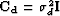, the fitting goal is(97)
with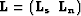and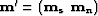.The normal equations are given by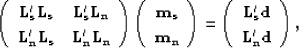(98)
whereand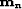are the unknowns. The least-square estimate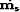ofcan be derived from the bottom row of equation (). The least-square estimate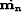ofcan be derived from the top row of equation (). We have, then,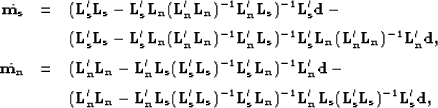(99) (100)
which can be simplified as follows: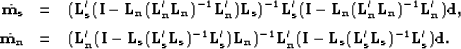(101) (102)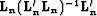is the coherent noise resolution matrix, whereasis the signal resolution matrix Tarantola (1987). Denotingandyields the following simplified expression forand: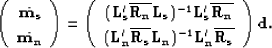(103)
By property of the resolution operators,andperform noise and signal filtering, i.e.,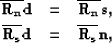(104)
if the noise and signal are well predicted by the noise and signal modeling operators. Nemeth (1996) demonstrates that the inverse of the Hessian in equation () is well conditioned if the noise and signal operators are orthogonal, meaning that they predict distinct parts of the model space without overlapping. If overlapping occurs, a model regularization term can improve the signal/noise separation.Next: Geometric interpretation of the Up: Least-squares solution of the Previous: Inversion of a 22
Stanford Exploration Project
5/5/2005